## Unit Circle: Sine and Cosine Functions

### Learning Outcomes

• Find function values for the sine and cosine of the special angles.
• Identify the domain and range of sine and cosine functions.
• Use reference angles to evaluate trigonometric functions.
• Evaluate sine and cosine values using a calculator.

To define our trigonometric functions, we begin by drawing a unit circle, a circle centered at the origin with radius 1, as shown in Figure 2. The angle (in radians) that $t$ intercepts forms an arc of length $s$. Using the formula $s=rt$, and knowing that $r=1$, we see that for a unit circle, $s=t$.

Recall that the x- and y-axes divide the coordinate plane into four quarters called quadrants. We label these quadrants to mimic the direction a positive angle would sweep. The four quadrants are labeled I, II, III, and IV.

For any angle $t$, we can label the intersection of the terminal side and the unit circle as by its coordinates, $\left(x,y\right)$. The coordinates $x$ and $y$ will be the outputs of the trigonometric functions $f\left(t\right)=\cos t$ and $f\left(t\right)=\sin t$, respectively. This means $x=\cos t$ and $y=\sin t$.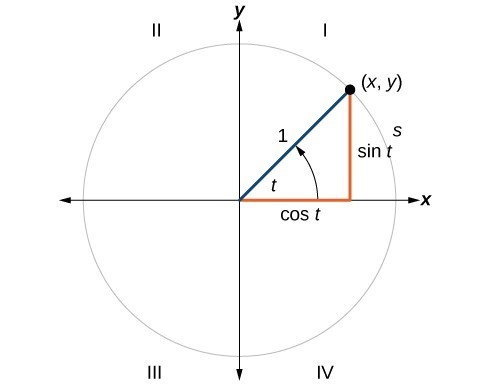Figure 2. Unit circle where the central angle is $t$ radians

### A General Note: Unit Circle

A unit circle has a center at $\left(0,0\right)$ and radius $1$ . In a unit circle, the length of the intercepted arc is equal to the radian measure of the central angle $1$.

Let $\left(x,y\right)$ be the endpoint on the unit circle of an arc of arc length $s$. The $\left(x,y\right)$ coordinates of this point can be described as functions of the angle.

## Defining Sine and Cosine Functions

Now that we have our unit circle labeled, we can learn how the $\left(x,y\right)$ coordinates relate to the arc length and angle. The sine function relates a real number $t$ to the y-coordinate of the point where the corresponding angle intercepts the unit circle. More precisely, the sine of an angle $t$ equals the y-value of the endpoint on the unit circle of an arc of length $t$. In Figure 2, the sine is equal to $y$. Like all functions, the sine function has an input and an output. Its input is the measure of the angle; its output is the y-coordinate of the corresponding point on the unit circle.

The cosine function of an angle $t$ equals the x-value of the endpoint on the unit circle of an arc of length $t$. In Figure 3, the cosine is equal to $x$.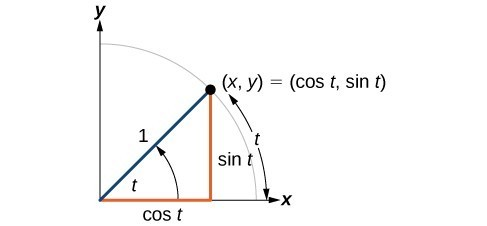Figure 3

Because it is understood that sine and cosine are functions, we do not always need to write them with parentheses: $\sin t$ is the same as $\sin \left(t\right)$ and $\cos t$ is the same as $\cos \left(t\right)$. Likewise, ${\cos }^{2}t$ is a commonly used shorthand notation for ${\left(\cos \left(t\right)\right)}^{2}$. Be aware that many calculators and computers do not recognize the shorthand notation. When in doubt, use the extra parentheses when entering calculations into a calculator or computer.

### A General Note: Sine and Cosine Functions

If $t$ is a real number and a point $\left(x,y\right)$ on the unit circle corresponds to an angle of $t$, then

$\cos t=x$
$\sin t=y$

### How To: Given a point P $\left(x,y\right)$ on the unit circle corresponding to an angle of $t$, find the sine and cosine.

1. The sine of $t$ is equal to the y-coordinate of point $P:\sin t=y$.
2. The cosine of $t$ is equal to the x-coordinate of point $P: \text{cos}t=x$.

### Example 1: Finding Function Values for Sine and Cosine

Point $P$ is a point on the unit circle corresponding to an angle of $t$, as shown in Figure 4. Find $\cos \left(t\right)$ and $\text{sin}\left(t\right)$.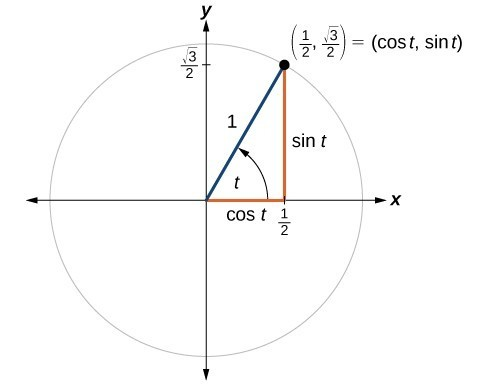Figure 4

### Try It

A certain angle $t$ corresponds to a point on the unit circle at $\left(-\frac{\sqrt{2}}{2},\frac{\sqrt{2}}{2}\right)$ as shown in Figure 5. Find $\cos t$ and $\sin t$.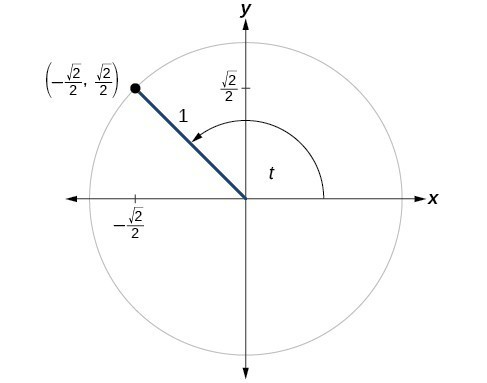Figure 5

## Finding Sines and Cosines of Angles on an Axis

For quadrantral angles, the corresponding point on the unit circle falls on the x- or y-axis. In that case, we can easily calculate cosine and sine from the values of $x$ and $y$.

### Example 2: Calculating Sines and Cosines along an Axis

Find $\cos \left(90^\circ \right)$ and $\text{sin}\left(90^\circ \right)$.

### Try It

Find cosine and sine of the angle $\pi$.

## The Pythagorean Identity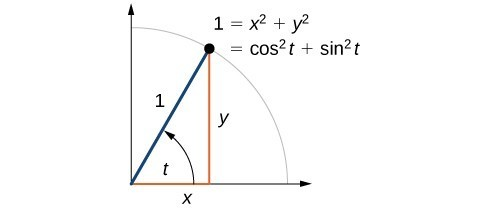Figure 7

Now that we can define sine and cosine, we will learn how they relate to each other and the unit circle. Recall that the equation for the unit circle is ${x}^{2}+{y}^{2}=1$. Because $x=\cos t$ and $y=\sin t$, we can substitute for $x$ and $y$ to get ${\cos }^{2}t+{\sin }^{2}t=1$. This equation, ${\cos }^{2}t+{\sin }^{2}t=1$, is known as the Pythagorean Identity.

We can use the Pythagorean Identity to find the cosine of an angle if we know the sine, or vice versa. However, because the equation yields two solutions, we need additional knowledge of the angle to choose the solution with the correct sign. If we know the quadrant where the angle is, we can easily choose the correct solution.

### A General Note: Pythagorean Identity

The Pythagorean Identity states that, for any real number $t$,

${\cos }^{2}t+{\sin }^{2}t=1$

### How To: Given the sine of some angle $t$ and its quadrant location, find the cosine of $t$.

1. Substitute the known value of $\sin \left(t\right)$ into the Pythagorean Identity.
2. Solve for $\cos \left(t\right)$.
3. Choose the solution with the appropriate sign for the x-values in the quadrant where $t$ is located.

### Example 3: Finding a Cosine from a Sine or a Sine from a Cosine

If $\sin \left(t\right)=\frac{3}{7}$ and $t$ is in the second quadrant, find $\cos \left(t\right)$.

### Try It

If $\cos \left(t\right)=\frac{24}{25}$ and $t$ is in the fourth quadrant, find $\sin\left(t\right)$.

## Finding Sines and Cosines of Special Angles

We have already learned some properties of the special angles, such as the conversion from radians to degrees. We can also calculate sines and cosines of the special angles using the Pythagorean Identity and our knowledge of triangles.

### Finding Sines and Cosines of 45° Angles

First, we will look at angles of $45^\circ$ or $\frac{\pi }{4}$, as shown in Figure 9. A $45^\circ -45^\circ -90^\circ$ triangle is an isosceles triangle, so the x- and y-coordinates of the corresponding point on the circle are the same. Because the x- and y-values are the same, the sine and cosine values will also be equal.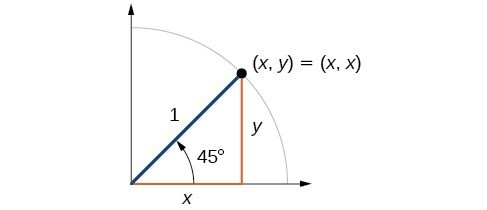Figure 9

At $t=\frac{\pi }{4}$ , which is 45 degrees, the radius of the unit circle bisects the first quadrantal angle. This means the radius lies along the line $y=x$. A unit circle has a radius equal to 1. So, the right triangle formed below the line $y=x$ has sides $x$ and $y\text{ }\left(y=x\right)$, and a radius = 1.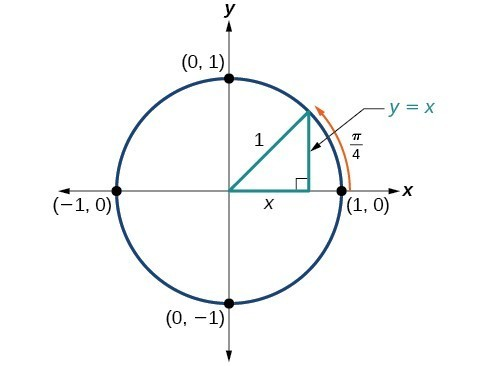Figure 10

From the Pythagorean Theorem we get

${x}^{2}+{y}^{2}=1$

Substituting $y=x$, we get

${x}^{2}+{x}^{2}=1$

Combining like terms we get

$2{x}^{2}=1$

And solving for $x$, we get

$\begin{gathered}{x}^{2}=\frac{1}{2}\\ x=\pm \frac{1}{\sqrt{2}}\end{gathered}$

In quadrant I, $x=\frac{1}{\sqrt{2}}$.

At $t=\frac{\pi }{4}$ or 45 degrees,

$\begin{gathered}\left(x,y\right)=\left(x,x\right)=\left(\frac{1}{\sqrt{2}},\frac{1}{\sqrt{2}}\right) \\ x=\frac{1}{\sqrt{2}},y=\frac{1}{\sqrt{2}}\\ \cos t=\frac{1}{\sqrt{2}},\sin t=\frac{1}{\sqrt{2}} \end{gathered}$

If we then rationalize the denominators, we get

\begin{align}\cos t&=\frac{1}{\sqrt{2}}\frac{\sqrt{2}}{\sqrt{2}} =\frac{\sqrt{2}}{2} \\ \sin t&=\frac{1}{\sqrt{2}}\frac{\sqrt{2}}{\sqrt{2}}=\frac{\sqrt{2}}{2} \end{align}

Therefore, the $\left(x,y\right)$ coordinates of a point on a circle of radius $1$ at an angle of $45^\circ$ are $\left(\frac{\sqrt{2}}{2},\frac{\sqrt{2}}{2}\right)$.

### Finding Sines and Cosines of 30° and 60° Angles

Next, we will find the cosine and sine at an angle of $30^\circ$, or $\frac{\pi }{6}$ . First, we will draw a triangle inside a circle with one side at an angle of $30^\circ$, and another at an angle of $-30^\circ$, as shown in Figure 11. If the resulting two right triangles are combined into one large triangle, notice that all three angles of this larger triangle will be $60^\circ$, as shown in Figure 12.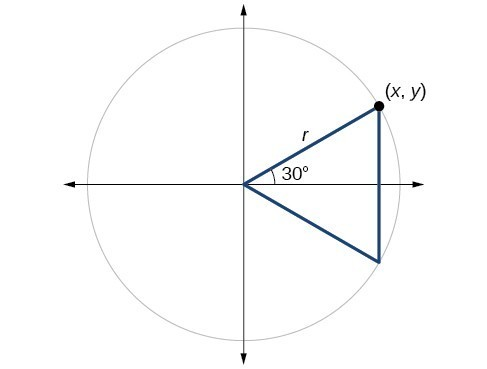Figure 11

Because all the angles are equal, the sides are also equal. The vertical line has length $2y$, and since the sides are all equal, we can also conclude that $r=2y$ or $y=\frac{1}{2}r$. Since $\sin t=y$ ,

$\sin \left(\frac{\pi }{6}\right)=\frac{1}{2}r$

And since $r=1$ in our unit circle,

\begin{align}\sin \left(\frac{\pi }{6}\right)=\frac{1}{2}\left(1\right)=\frac{1}{2}\end{align}

Using the Pythagorean Identity, we can find the cosine value.

$\begin{array}{cll}{\cos }^{2}\left(\frac{\pi }{6}\right)+{\sin }^{2}\left(\frac{\pi }{6}\right)=1 \hfill \\ {\cos }^{2}\left(\frac{\pi }{6}\right)+{\left(\frac{1}{2}\right)}^{2}=1 \\ {\cos }^{2}\left(\frac{\pi }{6}\right)=\frac{3}{4}&& \text{Use the square root property}. \\ \cos \left(\frac{\pi }{6}\right)=\frac{\pm \sqrt{3}}{\pm \sqrt{4}}=\frac{\sqrt{3}}{2}&& \text{Since }y\text{ is positive, choose the positive root}. \end{array}$

The $\left(x,y\right)$ coordinates for the point on a circle of radius $1$ at an angle of $30^\circ$ are $\left(\frac{\sqrt{3}}{2},\frac{1}{2}\right)$. At $t=\frac{\pi }{3}$ (60°), the radius of the unit circle, 1, serves as the hypotenuse of a 30-60-90 degree right triangle, $BAD$, as shown in Figure 13 below. Angle $A$ has measure $60^\circ$. At point $B$, we draw an angle $ABC$ with measure of $60^\circ$. We know the angles in a triangle sum to $180^\circ$, so the measure of angle $C$ is also $60^\circ$. Now we have an equilateral triangle. Because each side of the equilateral triangle $ABC$ is the same length, and we know one side is the radius of the unit circle, all sides must be of length 1.

The measure of angle $ABD$ is 30°. So, if double, angle $ABC$ is 60°. $BD$ is the perpendicular bisector of $AC$, so it cuts $AC$ in half. This means that $AD$ is $\frac{1}{2}$ the radius, or $\frac{1}{2}$. Notice that $AD$ is the x-coordinate of point $B$, which is at the intersection of the 60° angle and the unit circle. This gives us a triangle $BAD$ with hypotenuse of 1 and side $x$ of length $\frac{1}{2}$.

From the Pythagorean Theorem, we get

${x}^{2}+{y}^{2}=1$

Substituting $x=\frac{1}{2}$, we get

${\left(\frac{1}{2}\right)}^{2}+{y}^{2}=1$

Solving for $y$, we get

$\begin{gathered}\frac{1}{4}+{y}^{2}=1\\ {y}^{2}=1-\frac{1}{4}\\ {y}^{2}=\frac{3}{4}\\ y=\pm \frac{\sqrt{3}}{2}\end{gathered}$

Since $t=\frac{\pi }{3}$ has the terminal side in quadrant I where the y-coordinate is positive, we choose $y=\frac{\sqrt{3}}{2}\$, the positive value.

At $t=\frac{\pi }{3}$ (60°), the $\left(x,y\right)$ coordinates for the point on a circle of radius $1$ at an angle of $60^\circ$ are $\left(\frac{1}{2},\frac{\sqrt{3}}{2}\right)\$, so we can find the sine and cosine.

$\begin{gathered}\left(x,y\right)=\left(\frac{1}{2},\frac{\sqrt{3}}{2}\right) \\ x=\frac{1}{2},y=\frac{\sqrt{3}}{2}\\ \cos t=\frac{1}{2},\sin t=\frac{\sqrt{3}}{2} \end{gathered}$

We have now found the cosine and sine values for all of the most commonly encountered angles in the first quadrant of the unit circle. The table below summarizes these values.

 Angle 0 $\frac{\pi }{6}$, or 30° $\frac{\pi }{4}$, or 45° $\frac{\pi }{3}$, or 60° $\frac{\pi }{2}$, or 90° Cosine 1 $\frac{\sqrt{3}}{2}$ $\frac{\sqrt{2}}{2}$ $\frac{1}{2}$ 0 Sine 0 $\frac{1}{2}$ $\frac{\sqrt{2}}{2}$ $\frac{\sqrt{3}}{2}$ 1

Figure 14 shows the common angles in the first quadrant of the unit circle.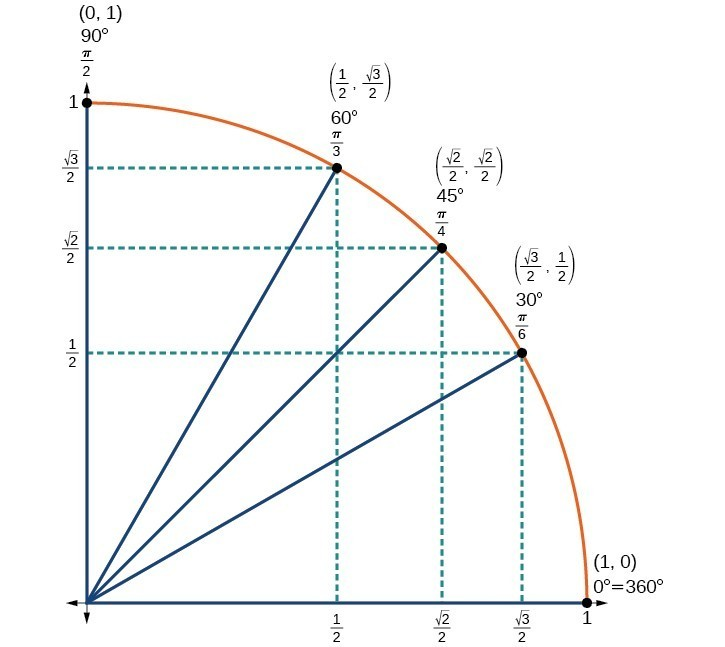Figure 14

### Using a Calculator to Find Sine and Cosine

To find the cosine and sine of angles other than the special angles, we turn to a computer or calculator. Be aware: Most calculators can be set into “degree” or “radian” mode, which tells the calculator the units for the input value. When we evaluate $\cos \left(30\right)$ on our calculator, it will evaluate it as the cosine of 30 degrees if the calculator is in degree mode, or the cosine of 30 radians if the calculator is in radian mode.

### How To: Given an angle in radians, use a graphing calculator to find the cosine.

1. If the calculator has degree mode and radian mode, set it to radian mode.
2. Press the COS key.
3. Enter the radian value of the angle and press the close-parentheses key “)”.
4. Press ENTER.

### Example 4: Using a Graphing Calculator to Find Sine and Cosine

Evaluate $\cos \left(\frac{5\pi }{3}\right)$ using a graphing calculator or computer.

### Try It

Evaluate $\sin \left(\frac{\pi }{3}\right)$.

## Identifying the Domain and Range of Sine and Cosine Functions

Now that we can find the sine and cosine of an angle, we need to discuss their domains and ranges. What are the domains of the sine and cosine functions? That is, what are the smallest and largest numbers that can be inputs of the functions? Because angles smaller than 0 and angles larger than $2\pi$ can still be graphed on the unit circle and have real values of $x,y$, and $r$, there is no lower or upper limit to the angles that can be inputs to the sine and cosine functions. The input to the sine and cosine functions is the rotation from the positive x-axis, and that may be any real number.

What are the ranges of the sine and cosine functions? What are the least and greatest possible values for their output? We can see the answers by examining the unit circle, as shown in Figure 15. The bounds of the x-coordinate are $\left[-1,1\right]$. The bounds of the y-coordinate are also $\left[-1,1\right]$. Therefore, the range of both the sine and cosine functions is $\left[-1,1\right]$.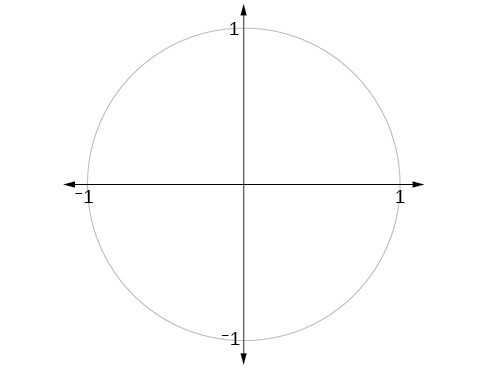Figure 15

We have discussed finding the sine and cosine for angles in the first quadrant, but what if our angle is in another quadrant? For any given angle in the first quadrant, there is an angle in the second quadrant with the same sine value. Because the sine value is the y-coordinate on the unit circle, the other angle with the same sine will share the same y-value, but have the opposite x-value. Therefore, its cosine value will be the opposite of the first angle’s cosine value.

Likewise, there will be an angle in the fourth quadrant with the same cosine as the original angle. The angle with the same cosine will share the same x-value but will have the opposite y-value. Therefore, its sine value will be the opposite of the original angle’s sine value.

As shown in Figure 16, angle $\alpha$ has the same sine value as angle $t$; the cosine values are opposites. Angle $\beta$ has the same cosine value as angle $t$; the sine values are opposites.

$\begin{array}{ccc}\sin \left(t\right)=\sin \left(\alpha \right)\hfill & \text{and}\hfill & \cos \left(t\right)=-\cos \left(\alpha \right)\hfill \\ \sin \left(t\right)=-\sin \left(\beta \right)\hfill & \text{and}\hfill & \cos \left(t\right)=\cos \left(\beta \right)\hfill \end{array}$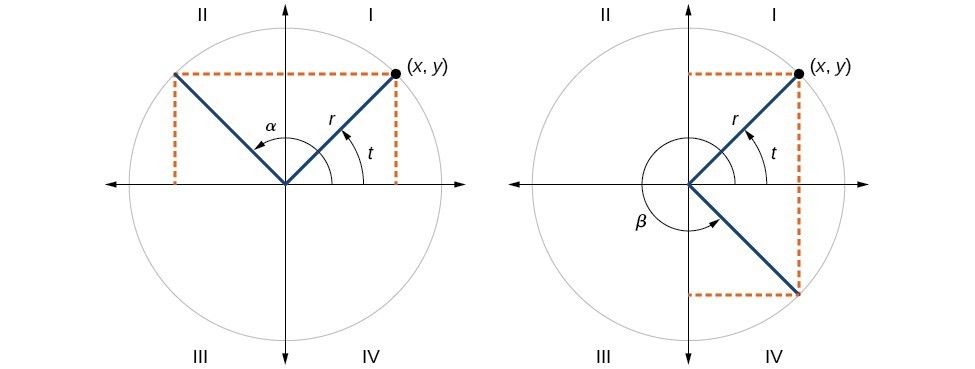Figure 16

Recall that an angle’s reference angle is the acute angle, $t$, formed by the terminal side of the angle $t$ and the horizontal axis. A reference angle is always an angle between $0$ and $90^\circ$, or $0$ and $\frac{\pi }{2}$ radians. As we can see from Figure 17, for any angle in quadrants II, III, or IV, there is a reference angle in quadrant I.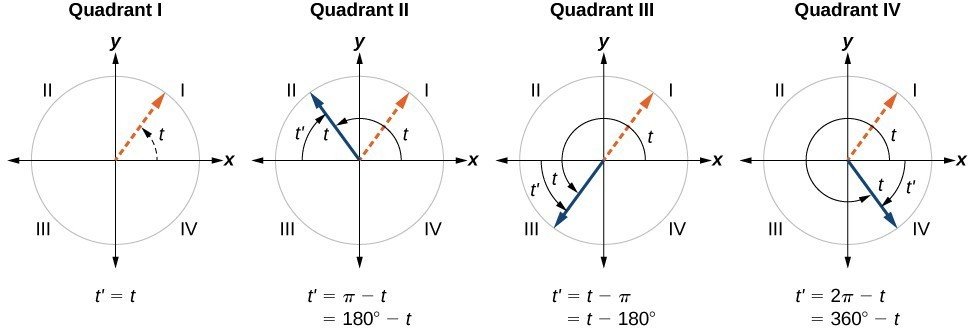Figure 17

### How To: Given an angle between $0$ and $2\pi$, find its reference angle.

1. An angle in the first quadrant is its own reference angle.
2. For an angle in the second or third quadrant, the reference angle is $|\pi -t|$ or $|180^\circ \mathrm{-t}|$.
3. For an angle in the fourth quadrant, the reference angle is $2\pi -t$ or $360^\circ \mathrm{-t}$.
4. If an angle is less than $0$ or greater than $2\pi$, add or subtract $2\pi$ as many times as needed to find an equivalent angle between $0$ and $2\pi$.

### Example 5: Finding a Reference Angle

Find the reference angle of $225^\circ$ as shown in Figure 18.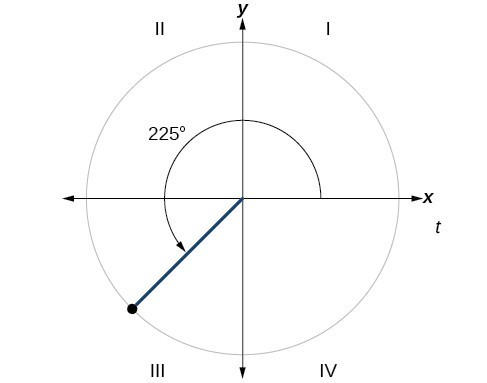Figure 18

### Try It

Find the reference angle of $\frac{5\pi }{3}$.

## Using Reference Angles

Now let’s take a moment to reconsider the Ferris wheel introduced at the beginning of this section. Suppose a rider snaps a photograph while stopped twenty feet above ground level. The rider then rotates three-quarters of the way around the circle. What is the rider’s new elevation? To answer questions such as this one, we need to evaluate the sine or cosine functions at angles that are greater than 90 degrees or at a negative angle. Reference angles make it possible to evaluate trigonometric functions for angles outside the first quadrant. They can also be used to find $\left(x,y\right)$ coordinates for those angles. We will use the reference angle of the angle of rotation combined with the quadrant in which the terminal side of the angle lies.

### Using Reference Angles to Evaluate Trigonometric Functions

We can find the cosine and sine of any angle in any quadrant if we know the cosine or sine of its reference angle. The absolute values of the cosine and sine of an angle are the same as those of the reference angle. The sign depends on the quadrant of the original angle. The cosine will be positive or negative depending on the sign of the x-values in that quadrant. The sine will be positive or negative depending on the sign of the y-values in that quadrant.

### A General Note: Using Reference Angles to Find Cosine and Sine

Angles have cosines and sines with the same absolute value as their reference angles. The sign (positive or negative) can be determined from the quadrant of the angle.

### How To: Given an angle in standard position, find the reference angle, and the cosine and sine of the original angle.

1. Measure the angle between the terminal side of the given angle and the horizontal axis. This is the reference angle.
2. Determine the values of the cosine and sine of the reference angle.
3. Give the cosine the same sign as the x-values in the quadrant of the original angle.
4. Give the sine the same sign as the y-values in the quadrant of the original angle.

### Example 6: Using Reference Angles to Find Sine and Cosine

1. Using a reference angle, find the exact value of $\cos \left(150^\circ \right)$ and $\text{sin}\left(150^\circ \right)$.
2. Using the reference angle, find $\cos \frac{5\pi }{4}$ and $\sin \frac{5\pi }{4}$.

### Try It

a. Use the reference angle of $315^\circ$ to find $\cos \left(315^\circ \right)$ and $\sin \left(315^\circ \right)$.

b. Use the reference angle of $-\frac{\pi }{6}$ to find $\cos \left(-\frac{\pi }{6}\right)$ and $\sin \left(-\frac{\pi }{6}\right)$.

### Using Reference Angles to Find Coordinates

Now that we have learned how to find the cosine and sine values for special angles in the first quadrant, we can use symmetry and reference angles to fill in cosine and sine values for the rest of the special angles on the unit circle. They are shown in Figure 19. Take time to learn the $\left(x,y\right)$ coordinates of all of the major angles in the first quadrant.

In addition to learning the values for special angles, we can use reference angles to find $\left(x,y\right)$ coordinates of any point on the unit circle, using what we know of reference angles along with the identities

$\begin{gathered}x=\cos t \\ y=\sin t \end{gathered}$

First we find the reference angle corresponding to the given angle. Then we take the sine and cosine values of the reference angle, and give them the signs corresponding to the y– and x-values of the quadrant.

### How To: Given the angle of a point on a circle and the radius of the circle, find the $\left(x,y\right)$ coordinates of the point.

1. Find the reference angle by measuring the smallest angle to the x-axis.
2. Find the cosine and sine of the reference angle.
3. Determine the appropriate signs for $x$ and $y$

### Example 7: Using the Unit Circle to Find Coordinates

Find the coordinates of the point on the unit circle at an angle of $\frac{7\pi }{6}$.

### Try It

Find the coordinates of the point on the unit circle at an angle of $\frac{5\pi }{3}$.

## Key Equations

 Cosine $\cos t=x$ Sine $\sin t=y$ Pythagorean Identity ${\cos }^{2}t+{\sin }^{2}t=1$

## Key Concepts

• Finding the function values for the sine and cosine begins with drawing a unit circle, which is centered at the origin and has a radius of 1 unit.
• Using the unit circle, the sine of an angle $t$ equals the y-value of the endpoint on the unit circle of an arc of length $t$ whereas the cosine of an angle $t$ equals the x-value of the endpoint.
• The sine and cosine values are most directly determined when the corresponding point on the unit circle falls on an axis.
• When the sine or cosine is known, we can use the Pythagorean Identity to find the other. The Pythagorean Identity is also useful for determining the sines and cosines of special angles.
• Calculators and graphing software are helpful for finding sines and cosines if the proper procedure for entering information is known.
• The domain of the sine and cosine functions is all real numbers.
• The range of both the sine and cosine functions is $\left[-1,1\right]$.
• The sine and cosine of an angle have the same absolute value as the sine and cosine of its reference angle.
• The signs of the sine and cosine are determined from the x– and y-values in the quadrant of the original angle.
• An angle’s reference angle is the size angle, $t$,
formed by the terminal side of the angle $t$ and the horizontal axis.
• Reference angles can be used to find the sine and cosine of the original angle.
• Reference angles can also be used to find the coordinates of a point on a circle.

## Glossary

cosine function
the x-value of the point on a unit circle corresponding to a given angle
Pythagorean Identity
a corollary of the Pythagorean Theorem stating that the square of the cosine of a given angle plus the square of the sine of that angle equals 1
sine function
the y-value of the point on a unit circle corresponding to a given angle
unit circle
a circle with a center at $\left(0,0\right)$